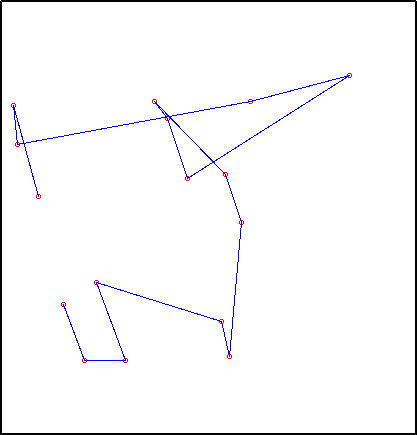## Traveling Salesman Problem by Genetic Algorithm

Here is another project for my artificial intelligence class. I wrote a generic genetic algorithm class in C++ and then applied that class to the traveling salesman problem. A genetic algorithm more tuned to the traveling salesman problem would work better.

This particular implementation can use up to 16 points defined in the weight matrix stored in travel.dat. This weight matrix can either be defined by hand or generated using gendat.m from a list of points stored in points.txt. A chromosome is 64 bits wide, which is 16 points with 4 bits each. To make sure that every possible chromosome is a valid solution, the points are selected out of a circular queue. Every 4 bits describes how far along the queue to walk before pulling out a point. With the circular queue, the chromosome could be as short as 50 bits, but I was trying different things and 64 bits is the simplest way to represent a solution. Here are some sample chromosomes:

``````1101100010100000011001010101010111000110101100111111000010111010, 9315
0000110100000001000010010101010111011000101100010110000010111010, 9339
1010001100010001010010011001010010100000101100011111000010111110, 9410
0101001000000011010010011001010011010100101011100111000111011110, 9355
0101001001000000010110100001010011010110101101000001000101000110, 9349
0101011100010011000010011011011011010101101100011111010010111110, 9311
0000111101000001000010011001010010110100101100011111100010000010, 9350
1000001111100000010110101011011011100000111101010111000010111010, 9428
0000111011000001000010010111011111111110101100010111000001000111, 9448
``````

The second number is the fitness value of the chromosome (10000 - path length). Below is a path found after 20000 iterations:It takes many iterations to find a reasonable solution and it never finds a really good solution. This is because each node in the chromosome depends on every single node before it. This is terrible for a genetic algorithm, but it’s really the best I could think of when using a generic genetic algorithm class like this.

A much better method would have the genetic algorithm actually know about the problem at hand, working with nodes rather than bits. Breeding would make cuts on nodes. Mutations would swap single nodes. Perhaps this can be written another time.